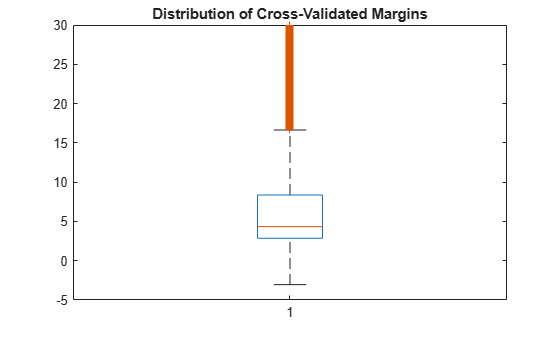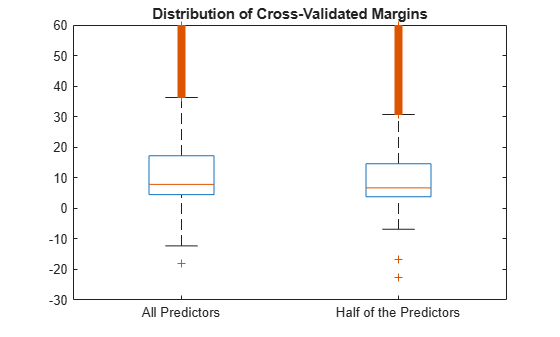# kfoldMargin

Classification margins for observations not used in training

## Syntax

``m = kfoldMargin(CVMdl)``

## Description

example

````m = kfoldMargin(CVMdl)` returns the cross-validated classification margins obtained by the cross-validated, binary, linear classification model `CVMdl`. That is, for every fold, `kfoldMargin` estimates the classification margins for observations that it holds out when it trains using all other observations.`m` contains classification margins for each regularization strength in the linear classification models that comprise `CVMdl`.```

## Input Arguments

expand all

Cross-validated, binary, linear classification model, specified as a `ClassificationPartitionedLinear` model object. You can create a `ClassificationPartitionedLinear` model using `fitclinear` and specifying any one of the cross-validation, name-value pair arguments, for example, `CrossVal`.

To obtain estimates, kfoldMargin applies the same data used to cross-validate the linear classification model (`X` and `Y`).

## Output Arguments

expand all

Cross-validated classification margins, returned as a numeric vector or matrix.

`m` is n-by-L, where n is the number of observations in the data that created `CVMdl` (see `X` and `Y`) and L is the number of regularization strengths in `CVMdl` (that is, `numel(CVMdl.Trained{1}.Lambda)`).

`m(i,j)` is the cross-validated classification margin of observation i using the linear classification model that has regularization strength `CVMdl.Trained{1}.Lambda(j)`.

Data Types: `single` | `double`

## Examples

expand all

`load nlpdata`

`X` is a sparse matrix of predictor data, and `Y` is a categorical vector of class labels. There are more than two classes in the data.

The models should identify whether the word counts in a web page are from the Statistics and Machine Learning Toolbox™ documentation. So, identify the labels that correspond to the Statistics and Machine Learning Toolbox™ documentation web pages.

`Ystats = Y == 'stats';`

Cross-validate a binary, linear classification model that can identify whether the word counts in a documentation web page are from the Statistics and Machine Learning Toolbox™ documentation.

```rng(1); % For reproducibility CVMdl = fitclinear(X,Ystats,'CrossVal','on');```

`CVMdl` is a `ClassificationPartitionedLinear` model. By default, the software implements 10-fold cross validation. You can alter the number of folds using the `'KFold'` name-value pair argument.

Estimate the cross-validated margins.

```m = kfoldMargin(CVMdl); size(m)```
```ans = 1×2 31572 1 ```

`m` is a 31572-by-1 vector. `m(j)` is the average of the out-of-fold margins for observation `j`.

Plot the k-fold margins using box plots.

```figure; boxplot(m); h = gca; h.YLim = [-5 30]; title('Distribution of Cross-Validated Margins')```One way to perform feature selection is to compare k-fold margins from multiple models. Based solely on this criterion, the classifier with the larger margins is the better classifier.

Load the NLP data set. Preprocess the data as in Estimate k-Fold Cross-Validation Margins.

```load nlpdata Ystats = Y == 'stats'; X = X';```

Create these two data sets:

• `fullX` contains all predictors.

• `partX` contains 1/2 of the predictors chosen at random.

```rng(1); % For reproducibility p = size(X,1); % Number of predictors halfPredIdx = randsample(p,ceil(0.5*p)); fullX = X; partX = X(halfPredIdx,:);```

Cross-validate two binary, linear classification models: one that uses the all of the predictors and one that uses half of the predictors. Optimize the objective function using SpaRSA, and indicate that observations correspond to columns.

```CVMdl = fitclinear(fullX,Ystats,'CrossVal','on','Solver','sparsa',... 'ObservationsIn','columns'); PCVMdl = fitclinear(partX,Ystats,'CrossVal','on','Solver','sparsa',... 'ObservationsIn','columns');```

`CVMdl` and `PCVMdl` are `ClassificationPartitionedLinear` models.

Estimate the k-fold margins for each classifier. Plot the distribution of the k-fold margins sets using box plots.

```fullMargins = kfoldMargin(CVMdl); partMargins = kfoldMargin(PCVMdl); figure; boxplot([fullMargins partMargins],'Labels',... {'All Predictors','Half of the Predictors'}); h = gca; h.YLim = [-30 60]; title('Distribution of Cross-Validated Margins')```The distributions of the margins of the two classifiers are similar.

To determine a good lasso-penalty strength for a linear classification model that uses a logistic regression learner, compare distributions of k-fold margins.

Load the NLP data set. Preprocess the data as in Estimate k-Fold Cross-Validation Margins.

```load nlpdata Ystats = Y == 'stats'; X = X';```

Create a set of 11 logarithmically-spaced regularization strengths from $1{0}^{-8}$ through $1{0}^{1}$.

`Lambda = logspace(-8,1,11);`

Cross-validate a binary, linear classification model using 5-fold cross-validation and that uses each of the regularization strengths. Optimize the objective function using SpaRSA. Lower the tolerance on the gradient of the objective function to `1e-8`.

```rng(10); % For reproducibility CVMdl = fitclinear(X,Ystats,'ObservationsIn','columns','KFold',5, ... 'Learner','logistic','Solver','sparsa','Regularization','lasso', ... 'Lambda',Lambda,'GradientTolerance',1e-8)```
```CVMdl = ClassificationPartitionedLinear CrossValidatedModel: 'Linear' ResponseName: 'Y' NumObservations: 31572 KFold: 5 Partition: [1×1 cvpartition] ClassNames: [0 1] ScoreTransform: 'none' Properties, Methods ```

`CVMdl` is a `ClassificationPartitionedLinear` model. Because `fitclinear` implements 5-fold cross-validation, `CVMdl` contains 5 `ClassificationLinear` models that the software trains on each fold.

Estimate the k-fold margins for each regularization strength.

```m = kfoldMargin(CVMdl); size(m)```
```ans = 1×2 31572 11 ```

`m` is a 31572-by-11 matrix of cross-validated margins for each observation. The columns correspond to the regularization strengths.

Plot the k-fold margins for each regularization strength. Because logistic regression scores are in [0,1], margins are in [-1,1]. Rescale the margins to help identify the regularization strength that maximizes the margins over the grid.

```figure boxplot(10000.^m) ylabel('Exponentiated test-sample margins') xlabel('Lambda indices')```Several values of `Lambda` yield k-fold margin distributions that are compacted near 10000. Higher values of lambda lead to predictor variable sparsity, which is a good quality of a classifier.

Choose the regularization strength that occurs just before the centers of the k-fold margin distributions start decreasing.

`LambdaFinal = Lambda(5);`

Train a linear classification model using the entire data set and specify the desired regularization strength.

```MdlFinal = fitclinear(X,Ystats,'ObservationsIn','columns', ... 'Learner','logistic','Solver','sparsa','Regularization','lasso', ... 'Lambda',LambdaFinal);```

To estimate labels for new observations, pass `MdlFinal` and the new data to `predict`.

expand all